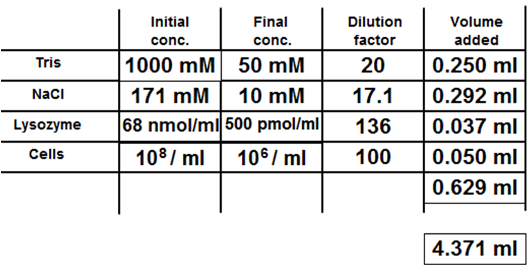#### You may also like### Golden Thoughts

Rectangle PQRS has X and Y on the edges. Triangles PQY, YRX and XSP have equal areas. Prove X and Y divide the sides of PQRS in the golden ratio.### At a Glance

The area of a regular pentagon looks about twice as a big as the pentangle star drawn within it. Is it?### Contact

A circular plate rolls in contact with the sides of a rectangular tray. How much of its circumference comes into contact with the sides of the tray when it rolls around one circuit?

# Mixed up Mixture

##### Age 14 to 16 Challenge Level:The solution is shown above, but is little help without the logical thought process which allowed its deduction.

Firstly, looking at the mass of data, it is notable that of the concentrations, two of them are given solely as a number per ml, rather than in moles per ml or l. These clearly match up with the 'cells' row, as it is common to talk about the number of cells per ml, rather than as a molar concentration. It is immediately clear that the $10^8$ must be the initial concentration and the $10^6$ value the final concentration, since a dilution occurs. This dilution factor is 100, as given by $\frac{10^8}{10^6}$. It is now possible to match these three pieces of data with the necessary 'volume added'. Dividing the total 5ml volume by the dilution factor gives an added volume of 0.050ml.

Matching the rest of the data is somewhat trickier, and it is perhaps useful to organise the data into three piles: concentrations, numbers, and volumes. Dilution factors can be coupled to pairs of concentrations through observation or trial and error. This reveals the two unused volumes, which can be put to one side.

It should hopefully be evident that Lysozyme is an enzyme, which is a protein formed from many amino acids. It thus has an exceptionally large RMM compared to either NaCl or Tris, and so its molar concentration is likely to be much smaller than either of these. Therefore, Lysozyme can be matched with the 68 nmol/ml, 500pmol/ml 136 and 0.037ml category.

Matching the final data to the Tris and NaCl is much trickier. One way of deducing this is to look up the cellular concentration of sodium ions, since the NaCl may be being added to the assay to ensure that the solution is isotonic, and so the cells will not lose or gain water by osmosis. Research reveals that these sodium ion concentrations are between 5 and 15 mMol, and so it is likely that data set with the final concentration of 10 mMol matches with NaCl.

Therefore, the final data set must match with Tris-Hcl!

The final part of the questions asks what the two unused volumes correspond to. 0.629ml corresponds to the total volume of the 4 solutions added so far to the assay, and 4.371ml corresponds to the amount of water that should be added to make up the solution to 5ml.